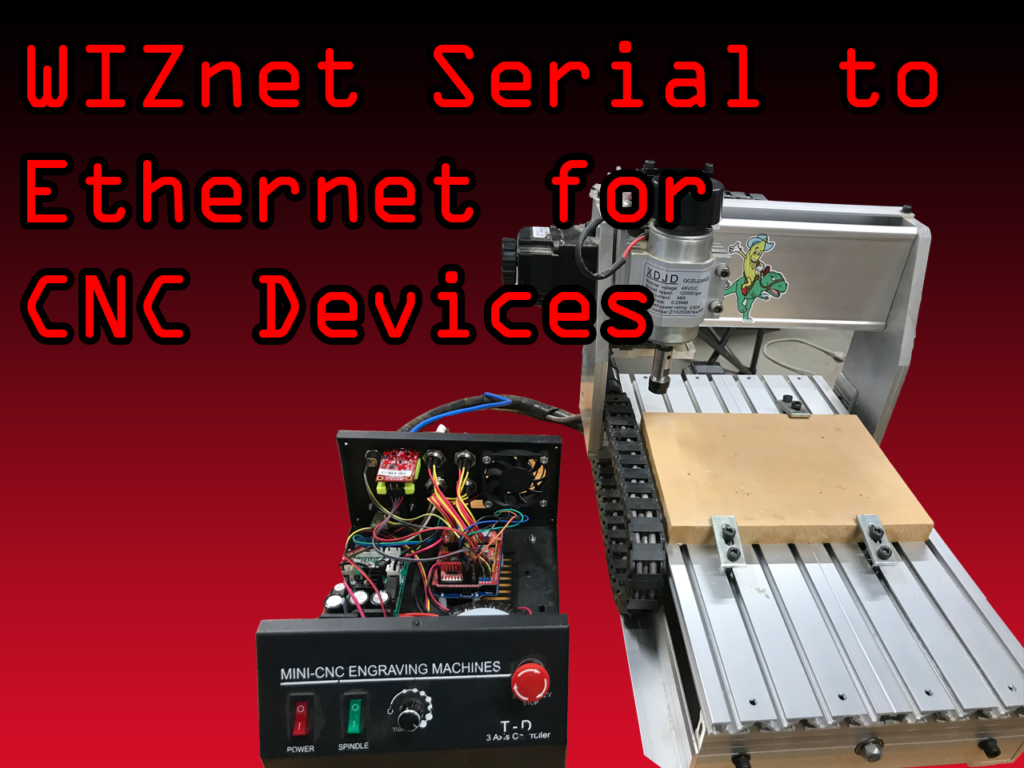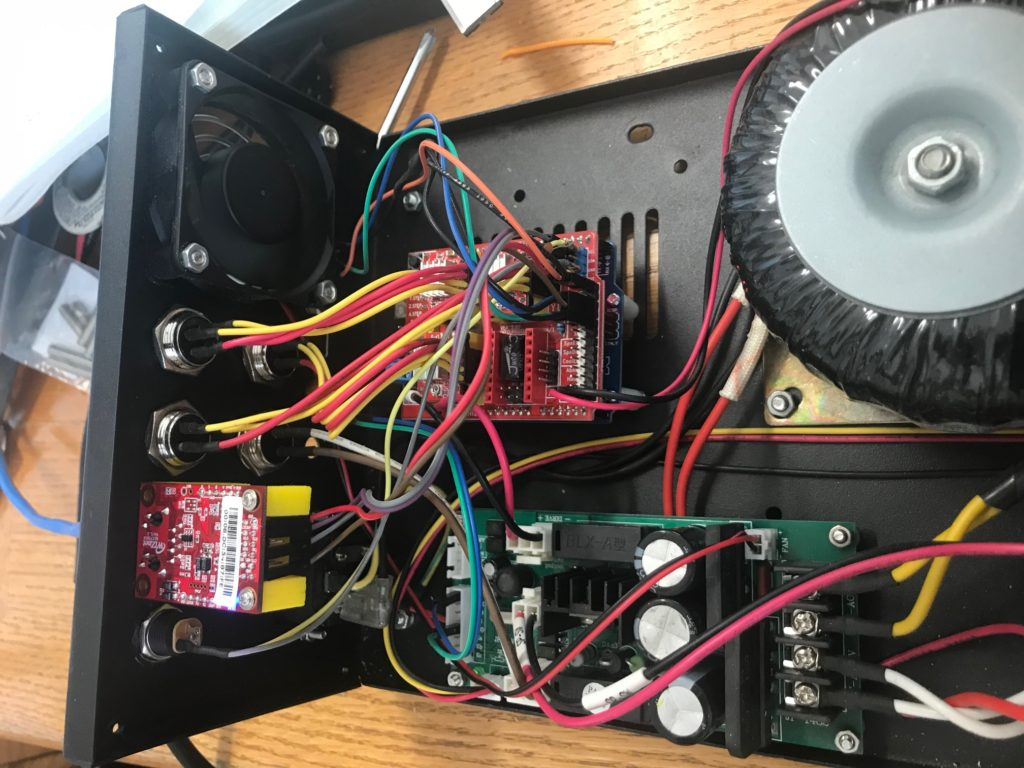# WIZnet WIZ750SR contest on Hackster.io submission

I finally completed my project for submission to the WIZnet Hackerster.io contest.

https://www.hackster.io/gmk/ethernet-connected-cnc-mill-or-other-machines-d0607aHere is the content of my GRBL configuration file :

```\$0=10 (step pulse, usec)
\$1=25 (step idle delay, msec)
\$4=0 (step enable invert, bool)
\$5=0 (limit pins invert, bool)
\$6=0 (probe pin invert, bool)
\$11=0.010 (junction deviation, mm)
\$12=0.002 (arc tolerance, mm)
\$13=0 (report inches, bool)
\$20=0 (soft limits, bool)
\$21=0 (hard limits, bool)
\$22=1 (homing cycle, bool)
\$24=25.000 (homing feed, mm/min)
\$25=500.000 (homing seek, mm/min)
\$26=250 (homing debounce, msec)
\$27=2.000 (homing pull-off, mm)
\$100=400.000 (x, step/mm)
\$101=400.000 (y, step/mm)
\$102=400.000 (z, step/mm)
\$110=500.000 (x max rate, mm/min)
\$111=500.000 (y max rate, mm/min)
\$112=250.000 (z max rate, mm/min)
\$120=10.000 (x accel, mm/sec^2)
\$121=10.000 (y accel, mm/sec^2)
\$122=10.000 (z accel, mm/sec^2)
\$130=196.000 (x max travel, mm)
\$131=280.000 (y max travel, mm)
\$132=100.000 (z max travel, mm)```